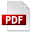# How tax affects your incomePDF version

Jack has an income of \$30,000 and Emily has an income of \$60,000. Calculate Jack and Emily’s income tax in each of the following situations.

Situation one – flat tax

Flat tax calculation: Jack

• Income: \$30,000
• Flat tax rate: 15%
• Calculate income tax
• Calculate income after paying tax

Flat tax calculation: Emily

• Income: \$60,000
• Flat tax rate: 15%
• Calculate income tax
• Calculate income after paying tax
Situation two – progressive tax

Progressive tax calculation: Jack

• Income: \$30,000
• Tax rate on the first \$20,000 of income: 15%
• Calculate the tax on the first \$20,000 of income
• Tax rate on income over \$20,000: 25%
• Calculate income tax on income over \$20,000
• Calculate total income tax
• Calculate income after paying tax

Progressive tax calculation: Emily

• Income: \$60,000.
• Tax rate on the first \$20,000 of income: 15%
• Calculate the tax on the first \$20,000 of income
• Tax rate on income over \$20,000: 25%
• Calculate income tax on income over \$20,000
• Calculate total income tax
• Calculate income after paying tax
Situation three – regressive tax

Regressive tax calculation: Jack

• Income: \$30,000
• Tax rate on the first \$20,000 of income: 15%
• Calculate the tax on the first \$20,000 of income
• Tax rate on income over \$20,000: 5%
• Calculate income tax on income over \$20,000
• Calculate total income tax
• Calculate income after paying tax

Regressive tax calculation: Emily

• Income: \$60,000
• Tax rate on the first \$20,000 of income: 15%
• Calculate the tax on the first \$20,000 of income
• Tax rate on income over \$20,000: 5%
• Calculate income tax on income over \$20,000
• Calculate total income tax
• Calculate income after paying tax